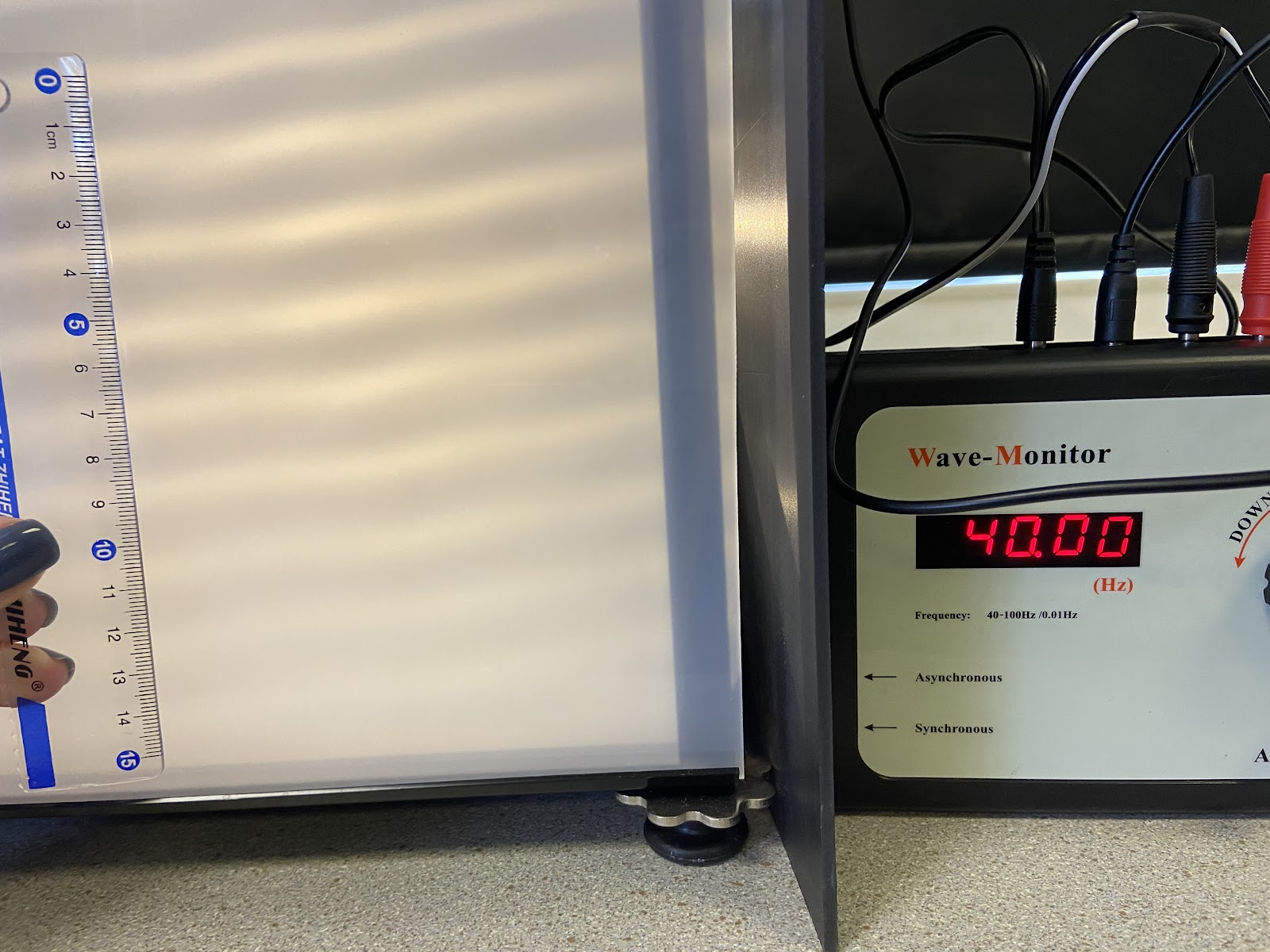frequency Hertz (Hz)

magnification No Unit (it is a ratio)

timeperiod second (s)

velocity metre/second (m/s)

wavelength metre (m)

distance metre(m)

speed  metre/second (m/s)

time seconds (s)

Waves transfer energy from one plane to another.

Waves can be:

• mechanical: they need a medium to trace through
• electromagnetic: the can travel in a vacuum without a medium

Waves may be either transverse or longitudinal. One whole wave is called an oscillation.

## Transverse Waves

The ripples on a water surface are an example of a transverse wave

The individual particles oscillate perpendicular to the direction of wave energy travel

## Longitudinal Waves

The ripples on a water surface are an example of a transverse wave.

Longitudinal waves show areas of compression and rarefaction.

Sound waves travelling through air are longitudinal.

The individual particles oscillate parallel to the direction of wave energy travel

For any mechanical wave, the individual particles that make up the wave do not travel far from their rest position. They just oscillate back and forth by plus or minus the amplitude of the wave.

in a longitudinal wave, the oscillations / vibrations are parallel to the direction of energy transfer
in a transverse wave, the oscillations / vibrations are perpendicular to the direction of energy transfer

The amplitude of a wave is the maximum displacement of a point on  a wave away from its undisturbed position.

The wavelength of a wave is the distance from a point on one wave  to the equivalent point on the adjacent wave.

The frequency of a wave is the number of waves passing a point  each second. Measured in Hertz, Hz.

The time period of a wave is how many seconds on wave takes to happen. It is 1/frequency.

The wave speed is the speed at which the energy is transferred (or  the wave moves) through the medium.

All waves obey the wave equation:

wave speed  = frequency × wavelength

wave speed  = frequency × wavelength

300 000 000 = frequency x 500

frequency = 300 000 000 / 500

The frequency of the wave is 600000 Hertz

wave speed  = frequency × wavelength

300 000 000 = 2 400 000 000 x wavelength

wavelength = 300 000 000 / 2 400 000 000

The wavelength is 0.125m

## Measuring the Speed of Sound Waves Air

Stand approximately 50m from a wall

Make a loud noise, start the timer when you make the noise

Stop the timer when you hear the echo

The speed of the sound is calculated using speed = distance/time

The distance the wave travels is 2 x the distance you are standing from the wall.

## Measuring the Speed of Waves on WaterUse a ripple tank screen to observe your waves.

The frequency of the waves being generated is read from the frequency generator.

The wavelength of the waves is found by measuring the length of ‘n’ waves and dividing the by ‘n’ (to improve accuracy)

The wave speed can be found using wave speed  = frequency × wavelength

In this example: Frequency = 40Hz

Length of 5 waves = 9cm = 0.09m

Length of 1 wave = 0.09/5 = 0.018 m

wave speed  = frequency × wavelength

wave speed = 40 x 0.018 = 0.072 m/s

• measure the distance between the student with the bricks and the wall with a trundle wheel or tape measure
• measure the time taken from banging the bricks to the echo
• double the measured distance to give the distance travelled
•  use:• repeat timings
• remove anomalies
• calculate a mean
• adjust the bar so that it just touches the surface of the water
• adjust motor to produce low frequency wave
• adjust the lamp until the pattern is seen clearly on the card underneath
• place a metre rule at right angles to the waves
• measure the length of a number of waves (minimum 3 waves)
• divide the length by the number of waves to give wavelength
• count the number of waves passing a point in a given time or read the frequency from the frequency generator
• divide the number of waves counted by the time to give the frequency

## Refraction

When a wave enters another medium the frequency stays the same but the speed and wavelength change. The wave will change direction because of the change in speed. This is observed as refraction.

If the wave slows down, the wavelength will decrease. If the wave speeds up, the wavelength will increase.